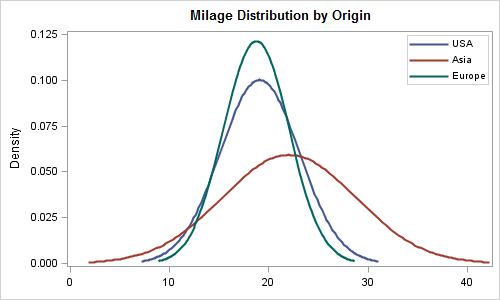## How to put two distribution curve together in Proc univariate

I use proc univaraiate to draw the distribution curve and histogram of two different groups of data by the following code:

Proc univariate data=data noprint;

histogram money /cfill=ligr normal cframe=liy barwidth=8 cv=black;

BY location;

RUN;

I would like to know how to put curves generated in the by statement together in one graph.

1 ACCEPTED SOLUTION

Accepted SolutionsJay54
Meteorite | Level 14

## How to put two distribution curve together in Proc univariate

This solution uses PROC SGPLOT's DENSITY statements.  Transform the data into multiple columns (one per class) and then use multiple density plots. I just used a data step with SASHELP.CARS to do that, but you can likely use proc transpose.

/*--Sort the data--*/

proc sort data=sashelp.cars out=cars;

by origin;

run;

/*--Separate the class data into multiple columns--*/

data cars1 cars2 cars3;

set cars (keep=mpg_city origin);

if origin='USA' then do; mpg_usa=mpg_city; output cars1; end;

if origin='Asia' then do; mpg_asia=mpg_city; output cars2; end;

if origin='Europe' then do; mpg_eur=mpg_city; output cars3; end;

run;

/*--Merge data into one data set--*/

data MpgByOrigin;

merge cars1(keep=mpg_usa) cars2(keep=mpg_asia) cars3(keep=mpg_eur);

run;

/*--Create graph using SGPLOT--*/

title 'Milage Distribution by Origin';

proc sgplot data=mpgbyorigin;

density mpg_usa / legendlabel='USA' lineattrs=(pattern=solid);

density mpg_asia  / legendlabel='Asia' lineattrs=(pattern=solid);

density mpg_eur  / legendlabel='Europe' lineattrs=(pattern=solid);

keylegend / location=inside position=topright across=1;

xaxis display=(nolabel);

run;

6 REPLIES 6

## How to put two distribution curve together in Proc univariate

You can get a COMPARATIVE panel of histograms by using the CLASS stmt instead of the BY stmt: CLASS location;

If this isn't sufficient, then I ask whether you just want the normal curves by themselves (in a single plot), or whether you want the densities fpor each group overlaid on a histogram.  If on a histogram, then are you asking for the histogram of ALL the data, across all groups?

## How to put two distribution curve together in Proc univariate

Actually, I just want the normal curves, how can I put into one graph to do the comparisonJay54
Meteorite | Level 14

## How to put two distribution curve together in Proc univariate

You can do this using SGPLOT procedure.  If this is what you need, I can attach the code.## How to put two distribution curve together in Proc univariate

yup

how to draw it?Jay54
Meteorite | Level 14

## How to put two distribution curve together in Proc univariate

This solution uses PROC SGPLOT's DENSITY statements.  Transform the data into multiple columns (one per class) and then use multiple density plots. I just used a data step with SASHELP.CARS to do that, but you can likely use proc transpose.

/*--Sort the data--*/

proc sort data=sashelp.cars out=cars;

by origin;

run;

/*--Separate the class data into multiple columns--*/

data cars1 cars2 cars3;

set cars (keep=mpg_city origin);

if origin='USA' then do; mpg_usa=mpg_city; output cars1; end;

if origin='Asia' then do; mpg_asia=mpg_city; output cars2; end;

if origin='Europe' then do; mpg_eur=mpg_city; output cars3; end;

run;

/*--Merge data into one data set--*/

data MpgByOrigin;

merge cars1(keep=mpg_usa) cars2(keep=mpg_asia) cars3(keep=mpg_eur);

run;

/*--Create graph using SGPLOT--*/

title 'Milage Distribution by Origin';

proc sgplot data=mpgbyorigin;

density mpg_usa / legendlabel='USA' lineattrs=(pattern=solid);

density mpg_asia  / legendlabel='Asia' lineattrs=(pattern=solid);

density mpg_eur  / legendlabel='Europe' lineattrs=(pattern=solid);

keylegend / location=inside position=topright across=1;

xaxis display=(nolabel);

run;

## How to put two distribution curve together in Proc univariate

One way is to transpose the data so that each category become a variable, and then use the SGPLOT DENSITY stmt.

To transpose the data, see http://blogs.sas.com/content/iml/2011/11/04/reshape-data-so-that-each-category-becomes-a-new-variabl...

Here's an example:

data combo;
keep Females Males;
merge sashelp.class(where=(sex="F") rename=(height=Females))
sashelp.class(where=(sex="M") rename=(height=Males));
run;

proc sgplot data=combo;
density Females / legendlabel="Female";
density Males / legendlabel="Male";
xaxis label="Height";
run;

Discussion stats
• 6 replies
• 2214 views
• 6 likes
• 3 in conversation